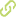A supervised learning approach to construct hyper-heuristics for constraint satisfaction Chapter in Scopus•
• Overview
•
• Identity
•
• Additional Document Info
•
• View All
•

Abstract

• Hyper-heuristics are methodologies that choose from a set of heuristics and decide which one to apply given some properties of the current instance. When solving a constraint satisfaction problem, the order in which the variables are selected to be instantiated has implications in the complexity of the search. In this paper we propose a logistic regression model to generate hyper-heuristics for variable ordering within constraint satisfaction problems. The first step in our approach requires to generate a training set that maps any given instance, expressed in terms of some of their features, to one suitable variable ordering heuristic. This set is used later to train the system and generate a hyper-heuristic that decides which heuristic to apply given the current features of the instances at hand at different steps of the search. The results suggest that hyper-heuristics generated through this methodology allow us to exploit the strengths of the heuristics to minimize the cost of the search. © 2013 Springer-Verlag Berlin Heidelberg.

Publication date

• November 29, 2013